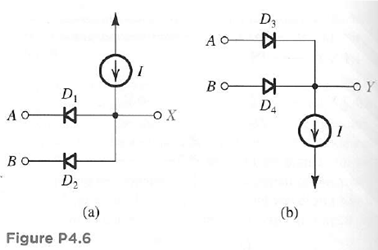# Ideal Diodes and Circuit Analysis

So today I started the problem set for my course on electronic circuits and the first item in the curriculum was ideal diodes.  Prior to the lesson I had already known that diodes only allowed the flow of current in one direction and blocked current flow in the other.  What I didn’t know were the analysis techniques used for these non-linear components.  While I was doing this problem set, it was surprising how much I had forgotten over the past eight months.

### Concept

I was given the circuit shown below (from Microelectronic Circuits 6th Edition by Sedra and Smith):I was asked for the voltage of the output terminal and the current flowing through the diode.  Finding the current was easy, but I didn’t quite get the voltage.  I thought to myself, “from what two points do I measure the potential difference?”, because after all, voltage measurements are always taken relative to two points.  It took me the longest time before I could just use ANY two points and the measure the voltage.

What I figured is that, for part a, since the diode is in forward bias, the diode will act as a short circuit.  I then can say that V – (-5) = 0, since the voltage difference along a short is 0.  From there, I can easily determine that V = -5.  I can apply that same logic to the other three.

### Nodal Analysis

I was given the circuit shown below (from Microelectronic Circuits 6th Edition by Sedra and Smith):From class, I remember the professor saying that since we cannot tell right away which diodes are on and off, we can only make an assumption about the states of the diodes and then apply the usual analytical techniques.  In the event that the result is unreasonable, we know that our assumption wrong.  I made my assumptions, but afterward, I didn’t know where to go next.  I had forgotten how to analyze these circuits.

Later, I realized that I could use nodal analysis.  Since I assumed the two diodes in part a were both on, I replaced them with short circuits.  I used the intersection right before the current splits as my node.  For part a, the current into the node was 3 V/5 kΩ = 0.6 mA.  The current leaving was some unknown current for diode 1, and a current for diode 2.  I could determine the current of diode 2 by taking the potential difference between the node and the -3 V output and dividing that by the resistance in the path.  The result was (0 – (-3))/ 10 kΩ = 0.3 mA.  From the nodal analysis equation 0.6 mA = current from diode 1 + 0.3 mA, the current from diode 1 is 0.3 mA.  And as for the voltage V, the potential difference between V and the -3 V output is equal to the voltage across the resistor, (0.3 mA)(10 kΩ) = V – (-3).  Therefore, V = 0.  Alternatively, I can use the potential difference between the node and the output, which equals zero, thus yielding the same result.

### Boolean Logic

The next issue I had was determining the Boolean outputs of a diode logic circuit.  Again, the picture below is from Electronic Circuits 6th Edition by Sedra and Smith.The issue I had here is that I did not know current sources have no voltage if there is no current running through them.  Looking back that sounded pretty intuitive.  Finding all possible combinations of inputs A and B for this logic circuit, we get the truth table below:

A B X Y
0 0 0 0
1 0 0 1
0 1 0 1
1 1 1 1

The outputs of X reveal an AND gate.  Setting A or B to a logic 0 in (a) will cause current to flow through that path.  The potential difference X – A or X – B (depending which one was set to 0), will yield 0, thus the output X will be zero as well.  When A and B are both set to logic 1, it causes the diodes to go into reverse bias, and node X will be powered by the current source.

The outputs of Y reveal an OR gate.  Setting A or B in (b) to have different inputs, either 1,0 or 0,1, will cause the diode to be in forward bias for the input that has the logic 1.  For the input with logic 0, the diode will in reverse bias.  Since one of the diodes is on, current will flow and output Y will yield a logic 1.  In the case of both diodes being off, no current will flow, thus causing output Y to yield logic 0.

### Other Notes

In the case of multiple parallel diodes in the same direction, if one of them is on, chances are they are all on (provided that the voltage across them is positive).  However, there may be a case where one parallel diode will want to flow into the other because of a greater voltage difference.  For example, if there were two parallel diodes, one with a 3V input and the other with a 2 V input both going to the same node, current from the 3 V input will want to flow into the diode connected to the 2 V input.  This will cause a reverse bias in the diode connected to the 2 V input, thus turning it off.

There is also another case where there are parallel diodes with different output voltages.  If current is flowing toward it, they will all be on, so they will all be a short circuit.  Which path will the current go to?  The path with the larger current.

This site uses Akismet to reduce spam. Learn how your comment data is processed.